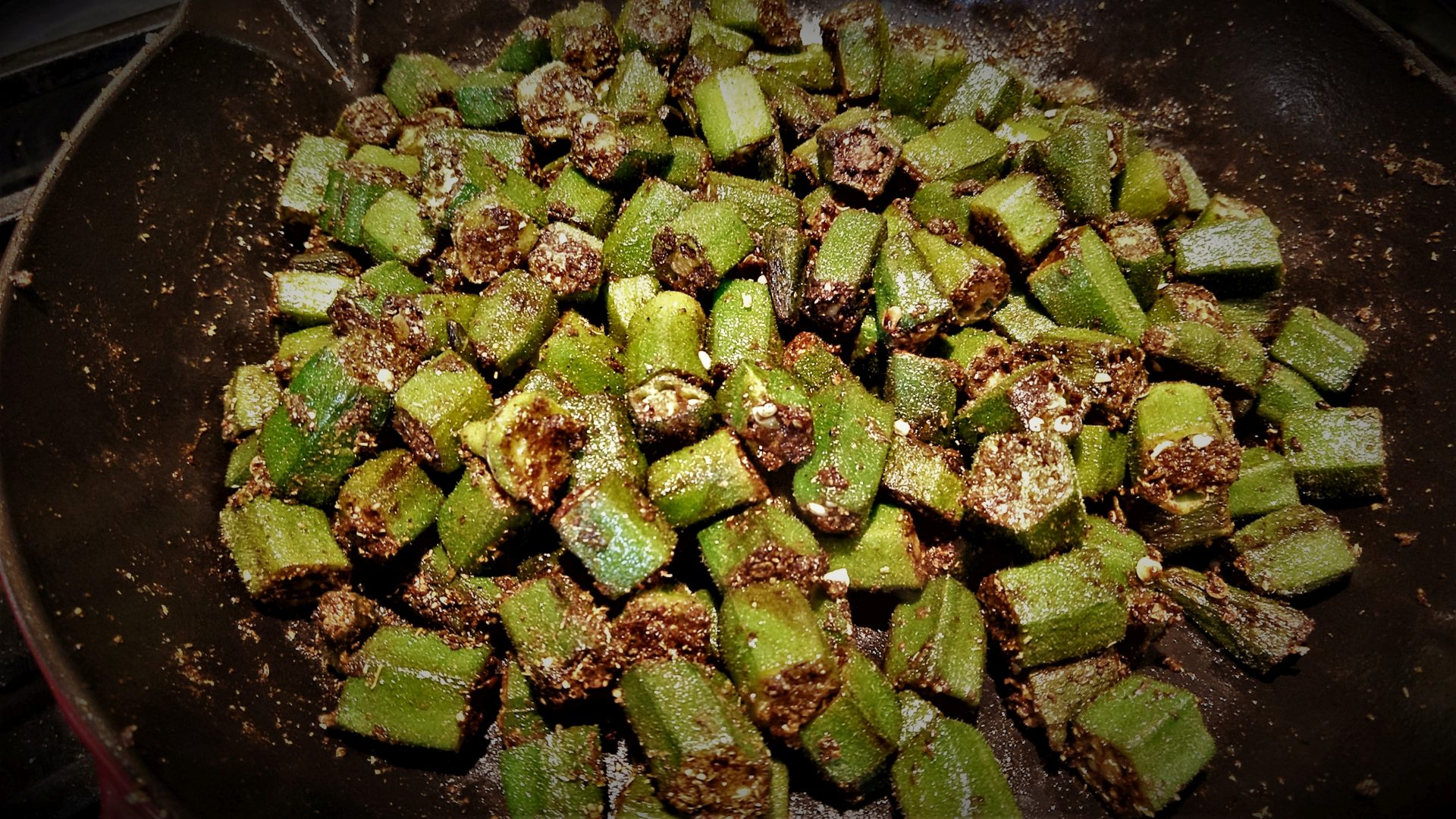# Making Touch Movement Controls Like Brawl Stars Using Phaser 3 while Learning Some Math

Here is a tutorial with code extracted from my son and my upcoming book – Intermediate JavaScript. This is the followup to my first book. This time we will teach you computer science and code concepts while building a game using the popular JavaScript game engine – Phaser 3. In this tutorial, I will walk through how we made touch controls for moving the blob in my son’s game BlobAttack. For the book, we are re-implementing the game using Phaser. Previously, he had implemented it by just moving HTML divs around the screen.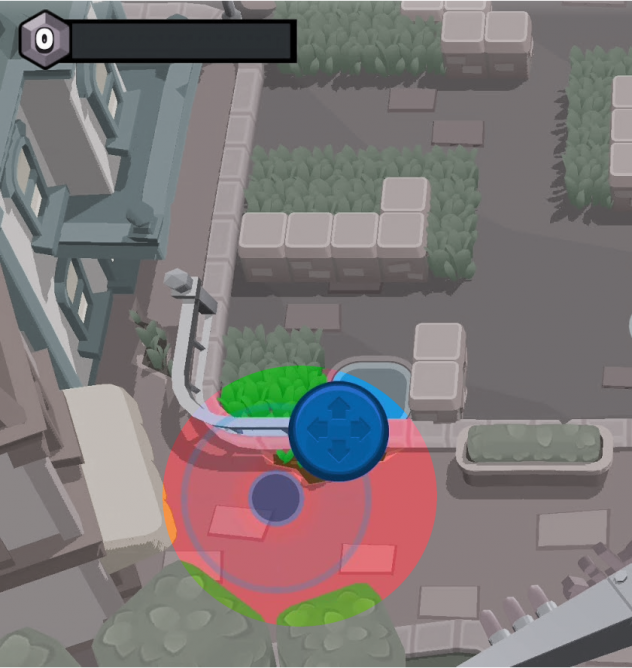For this version, my son insisted that we need to make touch controls like in one of his new favorite games – Brawl Stars. The basics are you drag around a small circle in a big circle and the player moves in whatever direction you are dragging the small circle in. See the highlighted area from the screenshot of Brawl Stars on the left.

My original plan was to have four simple buttons for up, down, right and left like the original BlobAttack, but being a glutton for punishment and wanting to take the opportunity to teach my son some Math, I agreed to this.

Even if this doesn’t make it to the final code in the book, at least it resulted in this blog post. Without further ado, let’s start.

To create the control, the first thing I had my son do is his favorite part – make the artwork. Once that is done, he is sucked in to complete the task even if it is hard. He uses paint.net, a free software for his game art. Here are the images he made for the controls: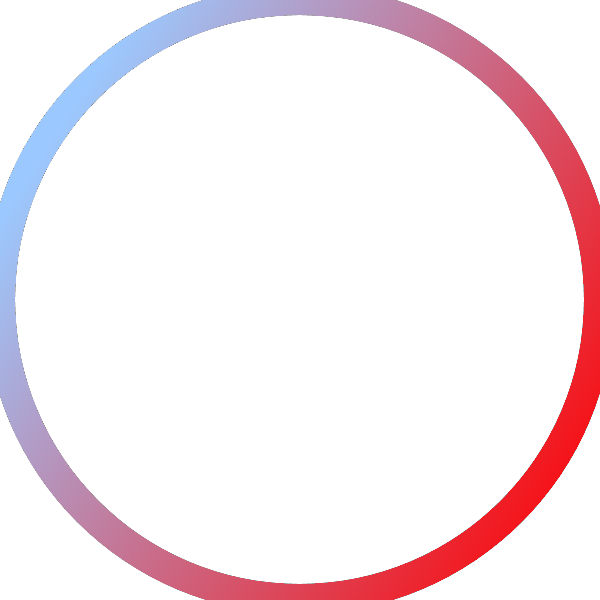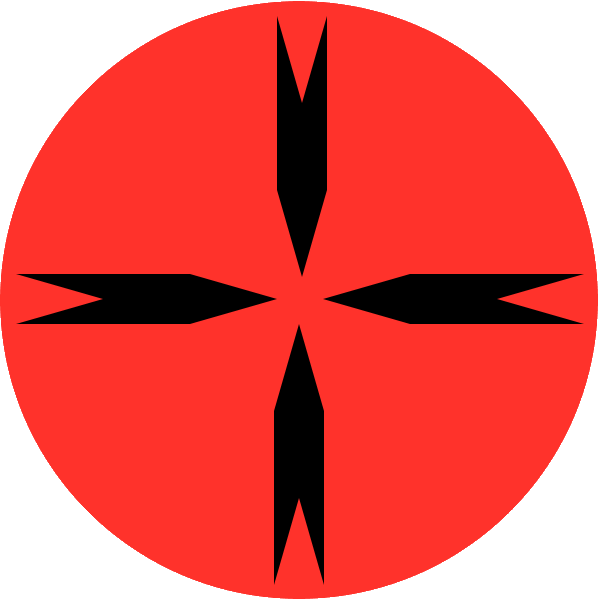Now for the code for the actual touch control:

First we position both the images where we need to. We will call the outside ring `phoneControlOuterRing` and the inner circle `phoneControlDragCircle`.

```// co-ordinates of the center of the phone control
let phoneControlX = game.height / 5 * 3 / 4
let phoneControlY = game.height - 3 / 4 * game.height / 5
let phoneControlCenter = {
x: phoneControlX,
y: phoneControlY
}

// create the outside ring for phone control
this.phoneControlOuterRing.displayWidth = game.height / 4
this.phoneControlOuterRing.displayHeight = game.height / 4

// create the middle draggable circle for phone control
this.phoneControlDragCircle.displayWidth = game.height / 8
this.phoneControlDragCircle.displayHeight = game.height / 8```

Next, we let Phaser know that the user can interact with the inner circle and make it draggable:

```// Allow user to interact with the phone control and drag it
this.phoneControlDragCircle.setInteractive()
game.input.setDraggable(this.phoneControlDragCircle)
```

Next we tell Phaser what to do when the user starts dragging and stops dragging:

```this.phoneControlDragCircle.on("drag", dragControl)
this.phoneControlDragCircle.on("dragend", returnToCenter)```

When the user starts dragging, Phaser will call a function called `dragControl` and when the user stops dragging, it will call a function called `returnToCenter`

When Phaser calls `dragControl`, it will send it three parameters – `pointer`, `dragX` and `dragY`. `dragX` and `dragY` are the the co-ordinates where the mouse has been dragged to. The `phoneControlDragCircle` cannot be dragged beyond the boundary of the `phoneControlOuterRing`. So the first thing we will do in the `dragControl` function is calculate the distance between the center of the control and the point where the mouse is dragged to:

```// the point where the control is dragged to
let draggedPoint = {
x: dragX,
y: dragY
}

// distance between the original center of the phone control and where it is dragged now
let d = Helper.distance(phoneControlCenter, draggedPoint)```

If the distance is less than or equal to the radius of the `phoneControlOuterRing`, we can move the `phoneControlDragCircle` to the dragged point. If the distance is greater than the radius of the `phoneControlOuterRing`, we need to calculate where to move the `phoneControlDragCircle` . See this diagram on how we do the calculation: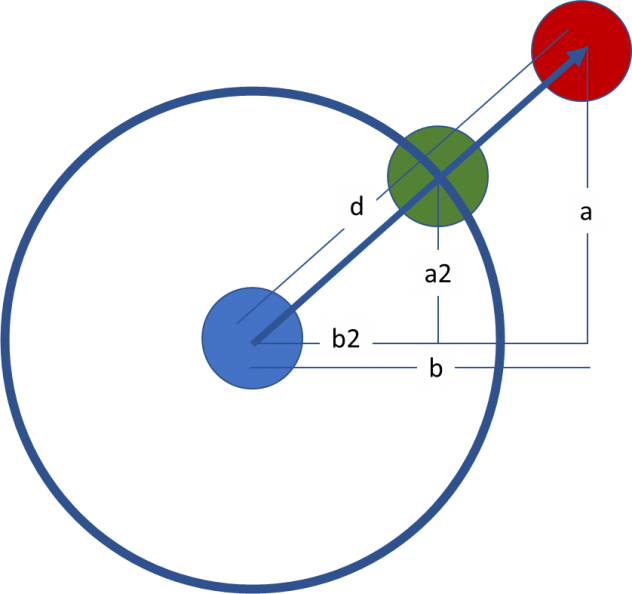The red circle is there the dragged point is. The green circle is where it should be and the blue circle represents the original position in the center.

We us the fact that the a reduces to a2 and b reduces to b2 in the same proportion as d reduces to the radius of the `phoneControlOuterRing`.

Here is the code to make that happen:

```// radius of the outer ring
let r = this.phoneControlOuterRing.displayWidth / 2

// a and b are the two sides of a right angled triangle where the hypotenuse is the line between phoneControlDragCircle Center and draggedPoint
let a = phoneControlCenter.y - dragY
let b = dragX - phoneControlCenter.x

// if the mouse tries to drag phoneControlDragCircle outside the phoneControlOuterRing then we should do our movements but not let phoneControlDragCircle leave the boundaries of phoneControlOuterRing
if(d > r) {
// calculate the proportional distance from the center to the edge of phoneControlOuterRing
let a2 = r * a / d
let b2 = r * b / d

// put phoneControlDragCircle at the edge of phoneControlOuterRing instead of outside
let X = phoneControlX + b2
let Y = phoneControlY - a2
this.phoneControlDragCircle.x = X
this.phoneControlDragCircle.y = Y
} else { //if phoneControlDragCircle is still inside phoneControlOuterRing
this.phoneControlDragCircle.x = dragX
this.phoneControlDragCircle.y = dragY
}```

Next we need to decide in which direction is the character moving. Here is the code to do that:

```// if b is bigger then go left or right, otherwise go up or down
if (Math.abs(b) > Math.abs(a)) {
// if b is negative, we are going left, otherwise we are going right.
if(b < 0) {
game.playerBlob.moveLeft()
} else if (b > 0) {
game.playerBlob.moveRight()
}
} else {
// if a is negative, we are going up, otherwise we are going down.
if(a > 0) {
game.playerBlob.moveUp()
} else if (a < 0 ) {
game.playerBlob.moveDown()
}
}```

Now let’s put all this together and see the entire function:

```// Called when the phone control is dragged
const dragControl = (pointer, dragX, dragY) => {
// the point where the control is dragged to
let draggedPoint = {
x: dragX,
y: dragY
}

// distance between the original center of the phone control and where it is dragged now
let d = Helper.distance(phoneControlCenter, draggedPoint)

// radius of the outer ring
let r = this.phoneControlOuterRing.displayWidth / 2

// a and b are the two sides of a right angled triangle where the hypotenuse is the line between phoneControlDragCircle Center and draggedPoint
let a = phoneControlCenter.y - dragY
let b = dragX - phoneControlCenter.x

// if the mouse tries to drag phoneControlDragCircle outside the phoneControlOuterRing then we should do our movements but not let phoneControlDragCircle leave the boundaries of phoneControlOuterRing
if(d > r) {
// calculate the proportional distance from the center to the edge of phoneControlOuterRing
let a2 = r * a / d
let b2 = r * b / d

// put phoneControlDragCircle at the edge of phoneControlOuterRing instead of outside
let X = phoneControlX + b2
let Y = phoneControlY - a2
this.phoneControlDragCircle.x = X
this.phoneControlDragCircle.y = Y
} else { //if phoneControlDragCircle is still inside phoneControlOuterRing
this.phoneControlDragCircle.x = dragX
this.phoneControlDragCircle.y = dragY
}

// if b is bigger then go left or right, otherwise go up or down
if (Math.abs(b) > Math.abs(a)) {
// if b is negative, we are going left, otherwise we are going right.
if(b < 0) {
game.playerBlob.moveLeft()
} else if (b > 0) {
game.playerBlob.moveRight()
}
} else {
// if a is negative, we are going up, otherwise we are going down.
if(a > 0) {
game.playerBlob.moveUp()
} else if (a < 0 ) {
game.playerBlob.moveDown()
}
}
}```

Next, let’s see the return to center function. That is very straightforward:

```// Return the phone control back to it's original position
const returnToCenter = () => {
game.playerBlob.stopMoving()
this.phoneControlDragCircle.x = phoneControlX
this.phoneControlDragCircle.y = phoneControlY
}```

Putting all this together, we have our `TouchControl` class:

```import Helper from './helper.js'

class TouchControl {
constructor(game) {
// co-ordinates of the center of the phone control
let phoneControlX = game.height / 5 * 3 / 4
let phoneControlY = game.height - 3 / 4 * game.height / 5
let phoneControlCenter = {
x: phoneControlX,
y: phoneControlY
}

// create the outside ring for phone control
this.phoneControlOuterRing.displayWidth = game.height / 4
this.phoneControlOuterRing.displayHeight = game.height / 4

// create the middle draggable circle for phone control
this.phoneControlDragCircle.displayWidth = game.height / 8
this.phoneControlDragCircle.displayHeight = game.height / 8

// Allow user to interact with the phone control and drag it
this.phoneControlDragCircle.setInteractive()
game.input.setDraggable(this.phoneControlDragCircle)

// Return the phone control back to it's original position
const returnToCenter = () => {
game.playerBlob.stopMoving()
this.phoneControlDragCircle.x = phoneControlX
this.phoneControlDragCircle.y = phoneControlY
}

// Called when the phone control is dragged
const dragControl = (pointer, dragX, dragY) => {
// the point where the control is dragged to
let draggedPoint = {
x: dragX,
y: dragY
}

// distance between the original center of the phone control and where it is dragged now
let d = Helper.distance(phoneControlCenter, draggedPoint)

// radius of the outer ring
let r = this.phoneControlOuterRing.displayWidth / 2

// a and b are the two sides of a right angled triangle where the hypotenuse is the line between phoneControlDragCircle Center and draggedPoint
let a = phoneControlCenter.y - dragY
let b = dragX - phoneControlCenter.x

// if the mouse tries to drag phoneControlDragCircle outside the phoneControlOuterRing then we should do our movements but not let phoneControlDragCircle leave the boundaries of phoneControlOuterRing
if(d > r) {
// calculate the proportional distance from the center to the edge of phoneControlOuterRing
let a2 = r * a / d
let b2 = r * b / d

// put phoneControlDragCircle at the edge of phoneControlOuterRing instead of outside
let X = phoneControlX + b2
let Y = phoneControlY - a2
this.phoneControlDragCircle.x = X
this.phoneControlDragCircle.y = Y
} else { //if phoneControlDragCircle is still inside phoneControlOuterRing
this.phoneControlDragCircle.x = dragX
this.phoneControlDragCircle.y = dragY
}

// if b is bigger then go left or right, otherwise go up or down
if (Math.abs(b) > Math.abs(a)) {
// if b is negative, we are going left, otherwise we are going right.
if(b < 0) {
game.playerBlob.moveLeft()
} else if (b > 0) {
game.playerBlob.moveRight()
}
} else {
// if a is negative, we are going up, otherwise we are going down.
if(a > 0) {
game.playerBlob.moveUp()
} else if (a < 0 ) {
game.playerBlob.moveDown()
}
}
}

this.phoneControlDragCircle.on("drag", dragControl)

this.phoneControlDragCircle.on("dragend", returnToCenter)
}
}

export default TouchControl```

Notice that we used something from the helper file to calculate distance (part of the Math learned). Here is the implementation of that:

```const Helper = {
/**
* Calculates the distance between two points
*/
distance: (point1, point2) =>  {
let x = point2.x - point1.x
let y = point2.y - point1.y
let getDistance = Math.sqrt(x*x + y*y)
return getDistance
}
}```

Now to use this TouchControl, in the create method for Phaser (see my previous tutorial on using Phase with ES6), just make a new object of the class:

`new TouchControl(this)`

To see this code in action, see our progress so far at blobattack 2.